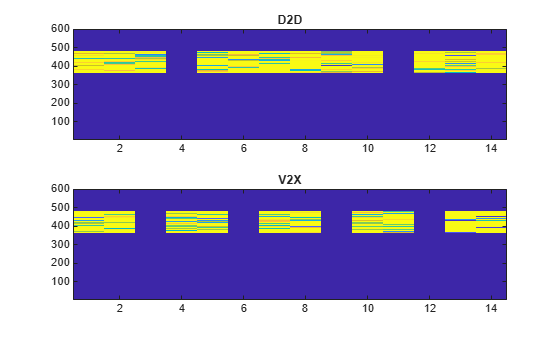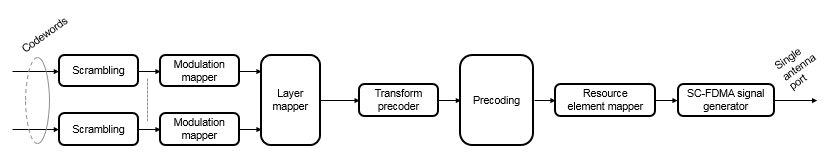# ltePSSCHIndices

PSSCH resource element indices

## Syntax

``[ind] = ltePSSCHIndices(ue)``
``````[ind,info] = ltePSSCHIndices(ue)``````
``[___] = ltePSSCHIndices(ue,opts)``

## Description

example

````[ind] = ltePSSCHIndices(ue)` returns a column vector of physical sidelink shared channel (PSSCH) resource element (RE) indices for the specified UE settings structure. By default, the indices are returned in one-based linear indexing form. You can use this form to directly index elements of a matrix representing the subframe resource grid for antenna port 1000. For more information, see Physical Sidelink Shared Channel Indexing.```

example

``````[ind,info] = ltePSSCHIndices(ue)``` also returns a structure containing PSSCH-related information for the specified UE settings structure. ```

example

````[___] = ltePSSCHIndices(ue,opts)` formats the returned indices using options specified by `opts`. This syntax supports output options from prior syntaxes.```

## Examples

collapse all

Write the complex PSSCH values into the PSSCH resource elements in a PSSCH subframe both for D2D normal cyclic prefix and V2X. Display an image of their locations to compare both sidelink modes. This mapping writes PSSCH values into the last SC-FDMA guard symbol within a subframe. The sidelink SC-FDMA modulator removes these values before transmission of the waveform.

Create a UE settings structure, an empty sidelink resource grid and D2D normal cyclic prefix. Define a PRB allocation, `ue.PRBSet`, with RB values from 30 to 39.

```ue = struct('NSLRB',50,'CyclicPrefixSL','Normal'); ue.NSAID = 1; ue.NSubframePSSCH = 1; ue.PRBSet = [30:39]'; ue.Modulation = 'QPSK'; subframe_D2D = lteSLResourceGrid(ue);```

Generate PSSCH indices. Populate the PSSCH resource elements in the subframe using a vector filled with zeros. For D2D normal cyclic prefix a PSSCH subframe contains (144 * `nprb` ) REs. The number of resource blocks is set to 10. Because the PSSCH uses QPSK modulation, there are 2 bits per symbol.

```pssch_indices = ltePSSCHIndices(ue); subframe_D2D(pssch_indices) = ltePSSCH(ue,zeros(2*10*144,1));```

Change user equipment settings to V2X sidelink mode. Set the V2X scrambling identity to 4567.

```ue.SidelinkMode = 'V2X'; ue.NXID = 4567; subframe_V2X = lteSLResourceGrid(ue); pssch_indices = ltePSSCHIndices(ue); subframe_V2X(pssch_indices) = ltePSSCH(ue,zeros(2*10*120,1));```

View the resource grid for both sidelink modes.

```subplot(2,1,1); image(400*abs(subframe_D2D)); axis xy; title('D2D'); subplot(2,1,2); image(400*abs(subframe_V2X)); axis xy; title(ue.SidelinkMode);```View the information structure output by the PSSCH resource element indexing function.

Create a UE settings structure.

```ue = struct('NSLRB',25,'CyclicPrefixSL','Normal','PRBSet',[5:22]', ... 'Modulation','16QAM');```

Generate PSSCH indices and the information structure. View the information structure to see the bit and symbol capacity of the PSSCH for this configuration.

```[pssch_indices,info] = ltePSSCHIndices(ue); info```
```info = struct with fields: G: 10368 Gd: 2592 ```

Compare PSSCH resource element indexing formats.

Create a UE settings structure.

`ue = struct('NSLRB',15,'CyclicPrefixSL','Normal','PRBSet',12);`

Generate PSSCH indices using one-based linear indexing (default), zero-based linear indexing, and one-based subscript row style.

One-based linear indexing

```pssch_indices = ltePSSCHIndices(ue); pssch_indices(1)```
```ans = uint32 145 ```

Zero-based linear indexing

```opts = '0based'; pssch_indices_0based = ltePSSCHIndices(ue,opts); pssch_indices_0based(1)```
```ans = uint32 144 ```

For zero-based indexing, the first assigned index is one lower than the one-based indexing.

One-based indexing in `[subcarrier,symbol,port]` subscript row style

Inspect the unique symbol values to see which symbols are occupied by the PSSCH.

```opts = {'sub' '1based'}; pssch_indices_sub = ltePSSCHIndices(ue,opts); unique(pssch_indices_sub(:,2,:))```
```ans = 12x1 uint32 column vector 1 2 3 5 6 7 8 9 10 12 ⋮ ```

Only the symbols 4 and 11 are not occupied. For one-based indexing, these two PSSCH subframe symbols are always reserved for transmission of the PSSCH DM-RS.

## Input Arguments

collapse all

User equipment settings, specified as a parameter structure containing these fields:

Sidelink mode, specified as `'D2D'` or `'V2X'`.

Data Types: `char` | `string`

Number of sidelink resource blocks, specified as an integer scalar from 6 to 110.

Example: `6`, which corresponds to a channel bandwidth of 1.4 MHz.

Data Types: `double`

Cyclic prefix length, specified as `'Normal'` or `'Extended'`.

Data Types: `char` | `string`

Zero-based physical resource block (PRB) indices, specified as an integer column vector or a two-column integer matrix.

The PSSCH is intended to be transmitted in the same PRB in each slot of a subframe. Therefore, specifying `PRBSet` as a single column of PRB indices is recommended. However, for a nonstandard slot-hopping PRB allocation, `PRBSet` can be specified as a two-column matrix of indices corresponding to slot-wise resource allocations for PSSCH.

Data Types: `double`

Modulation type, specified as `'QPSK'` or `'16QAM'`. Only required when the `info` output is assigned. `Modulation` is used to set the `info`.`G` output field.

Data Types: `char` | `string`

Data Types: `struct`

Output format options for resource element indices, specified as a character vector, cell array of character vectors, or string array. For convenience, you can specify several options as a single character vector or string scalar by a space-separated list of values placed inside the quotes. Values for `opts` when specified as a character vector include (use double quotes for string) :

Category Options Description

Indexing style

`'ind'` (default)

The returned indices are in linear index style.

`'sub'`

The returned indices are in `[subcarrier,symbol,port]` subscript row style.

Index base

`'1based'` (default)

The returned indices are one-based.

`'0based'`

The returned indices are zero-based.

Example: `'ind 1based'`, `"ind 1based"`, `{'ind','1based'}`, or `["ind","1based"]` specify the same formatting options.

Data Types: `char` | `string` | `cell`

## Output Arguments

collapse all

PSSCH resource element indices, returned as an integer column vector or a three-column integer matrix. The returned vector or matrix has NPRB × 144 PSSCH resource element indices for D2D normal cyclic prefix or NPRB × 120 PSSCH resource element indices for D2D extended cyclic prefix and V2X. NPRB is the number of physical resource blocks (PRB) used for transmission. For more information, see Physical Sidelink Shared Channel Indexing and Physical Sidelink Shared Channel Processing.

PSSCH subframe resource information, returned as a structure containing these fields:

PSSCH bit capacity, returned as an integer. For more information, see Physical Sidelink Shared Channel Processing.

PSSCH symbol capacity, returned as an integer. The number of PSSCH resource elements (NRE) in a subframe. For more information, see Physical Sidelink Shared Channel Processing.

Data Types: `struct`

collapse all

### Physical Sidelink Shared Channel Indexing

Use the `ltePSSCHIndices` function and the corresponding `ltePSSCH` sequence function to populate the PSSCH subframe resource grid. The PSSCH is transmitted in the available SC-FDMA symbols in a PSSCH subframe, using a single layer on antenna port 1000. It excludes each symbol per slot assigned to PSSCH DM-RS. For more information on PSSCH DM-RS, see the `ltePSSCHDRSIndices` function. The indices are ordered as the PSSCH modulation symbols should be mapped, applying frequency-first mapping. The resource elements in the last SC-FDMA symbol within a subframe are counted in the mapping process but should not be transmitted. The sidelink-specific SC-FDMA modulation creates this guard symbol. For more information on mapping symbols to the resource element grid, see Resource Grid Indexing.

### Physical Sidelink Shared Channel Processing

Physical sidelink shared channel (PSSCH) processing includes PSSCH-specific scrambling, QPSK or 16-QAM modulation, and SC-FDMA transform precoding. PSSCH processing follows the processing steps used for PUSCH, with variations defined in TS 36.211, Section 9.3.For PSSCH, the input codeword length is Mbits = NRE × Nbps, where Nbps is the number of bits per symbol. PSSCH modulation is either QPSK (2 bits per symbol) or 16 QAM (4 bits per symbol).

The number of PSSCH resource elements (NRE) in a subframe is NRE = NPRB × NREperPRB × NSYM and includes symbols associated with the sidelink SC-FDMA guard symbol.

• NPRB is the number of physical resource blocks (PRB) used for transmission.

• NREperPRB is the number of resource elements in a PRB. Each PRB has 12 resource elements.

• NSYM is the number of SC-FDMA symbols in a PSSCH subframe, including symbols associated with the sidelink SC-FDMA guard symbol. The number of SC-FDMA symbols in a PSSCH subframe is 12 for D2D normal cyclic prefix or 10 for D2D extended cyclic prefix and V2X.

The `info` structure output by `ltePSSCHIndices` provides Mbits and NRE as `info.G` and `info.Gd` respectively.

The scrambling sequence generator is initialized with ${c}_{\text{init}}={n}_{\text{ID}}^{\text{X}}×{2}^{14}+{n}_{\text{ssf}}^{\text{PSSCH}}×{2}^{9}+510$ at the start of every PSSCH subframe. For D2D sidelink, ${n}_{\text{ID}}^{\text{SA}}$ is the destination identity (`NSAID`) obtained from the sidelink shared channel. For V2X, ${n}_{\text{ID}}^{\text{SA}}$ is the V2X scrambling identity (`NXID`). ${n}_{\text{ssf}}^{\text{PSSCH}}$ is the subframe number in the PSSCH subframe pool (`NSubframePSSCH`).

`ltePSSCH` requires `CyclicPrefixSL` to deduce the number of resource blocks allocated for SC-FDMA precoding symbols.

 3GPP TS 36.211. “Evolved Universal Terrestrial Radio Access (E-UTRA); Physical Channels and Modulation.” 3rd Generation Partnership Project; Technical Specification Group Radio Access Network. URL: https://www.3gpp.org.